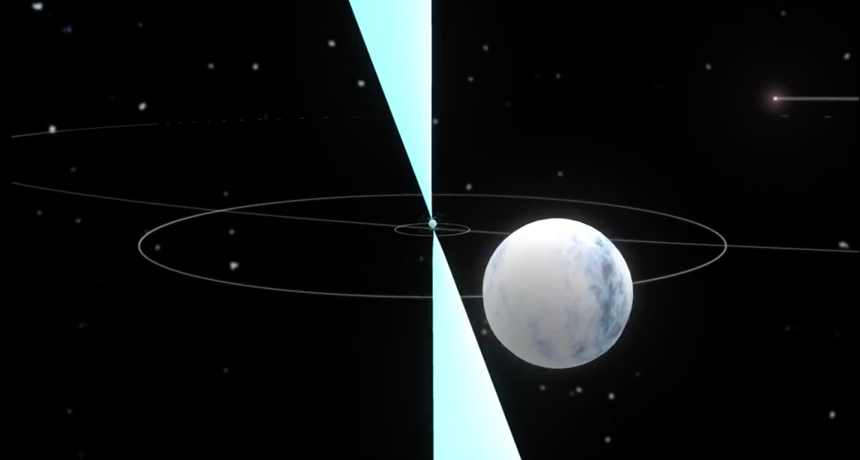# Testing Einstein’s Theory With A Triple Play

In Gravity by Brian Koberlein3 Comments

Einstein’s theory of gravity has been tested in lots of ways, from the slow precession of Mercury’s orbit, to the detection of gravitational waves. So far the theory has passed every test, but that doesn’t necessarily mean it’s completely true. Like any theory, general relativity is based upon certain assumptions about the way the universe works. The biggest assumption in relativity is the principle of general equivalence

The equivalence principle was proposed by both Galileo and Newton, and basically states that any two objects will fall at the same rate under gravity. Barring things like air resistance, a bowling ball and a feather should fall at the same rate. Experiments that have tested the principle of equivalence show it’s a good approximation at the very least.

In Newtonian gravity, this just means that the gravitational force on an object is proportional to its mass, so even if the equivalence principle is only an approximation we could still use Newtonian gravity. But Einstein’s theory of relativity, gravity isn’t a force, but simply an effect of the warp and weft of spacetime. In order for this to be true, the equivalence principle can’t be approximately true, it has to be exactly true. If objects “fall” due to the bending of space itself, then everything must fall at the same rate, because they are all in the same spacetime.

But there’s an interesting twist to this principle. One of the things relativity predicts is that mass and energy are related. This is where Einstein’s most famous equation, E = mc2, comes into play. Normally the “relativistic mass” of an object is effectively the same as its regular mass, but objects like neutron stars have such strong gravitational and electromagnetic fields that their relativistic mass is a bit larger than the mass of their matter alone. If the gravitational force on an object is proportional to its mass-energy, then a neutron star should fall slightly faster than lighter objects. If Einstein is right, then a neutron star should fall at exactly the same rate as anything else.

A few years ago, astronomers discovered a system of three stars orbiting closely together. Two of them are white dwarf stars, while the third is a neutron star. The neutron star is also a pulsar, which means it emits regular pulses of radio energy. The timing of these pulses are determined by the rotation of the neutron star, which is basically constant. Any variation in the timing of the pulses is therefore due to the motion of the neutron star in its orbit. In other words, we can use the radio pulses to measure the motion of the neutron star very precisely.

Each of the stars in this system is basically “falling” in the gravitational field of the others. Recently a team of astronomers observed this system to see if the neutron star falls at a different rate different from Einstein’s prediction. Their result agreed with Einstein. To within 0.16 thousandths of a percent (the observational limit of their data) the neutron star falls at the same rate as a white dwarf.

Once again, Einstein’s gravitational theory is right.

Paper: A. Archibald et al. Testing general relativity with a millisecond pulsar in a triple system. 231st meeting of the American Astronomical Society, Oxon Hill, Md. (2018)

1.1.Author
2.